# envspectrum

Envelope spectrum for machinery diagnosis

## Syntax

``es = envspectrum(x,fs)``
``es = envspectrum(xt)``
``es = envspectrum(___,Name,Value)``
``[es,f,env,t] = envspectrum(___)``
``envspectrum(___)``

## Description

example

````es = envspectrum(x,fs)` returns the envelope spectrum of a signal `x` sampled at a rate `fs`. If `x` is a matrix, then the function computes the envelope spectrum independently for each column and returns the result in the corresponding column of `es`.```

example

````es = envspectrum(xt)` returns the envelope spectrum of a signal stored in the MATLAB® timetable `xt`.```

example

````es = envspectrum(___,Name,Value)` specifies additional options for any of the previous syntaxes using name-value pair arguments. Options include the algorithm used to compute the envelope signal and the frequency band over which to estimate the spectrum.```
````[es,f,env,t] = envspectrum(___)` returns `f`, a vector of frequencies at which `es` is computed; `env`, the envelope signal; and `t`, the times at which `env` is computed.```
````envspectrum(___)` with no output arguments plots the envelope signal and the envelope spectrum in the current figure.```

## Examples

collapse all

Simulate two vibration signals, one from a healthy bearing and one from a damaged bearing. Compute and compare their envelope spectra.

A bearing with a pitch diameter of 12 cm has eight rolling elements. Each rolling element has a diameter of 2 cm. The outer race remains stationary as the inner race is driven at 25 cycles per second. An accelerometer samples the bearing vibrations at 10 kHz.

```fs = 10000; f0 = 25; n = 8; d = 0.02; p = 0.12;```The vibration signal from the healthy bearing includes several orders of the driving frequency. Plot 0.1 second of data.

```t = 0:1/fs:1-1/fs; z = [1 0.5 0.2 0.1 0.05]*sin(2*pi*f0*[1 2 3 4 5]'.*t)/5; plot(t,z) xlim([0.4 0.5])```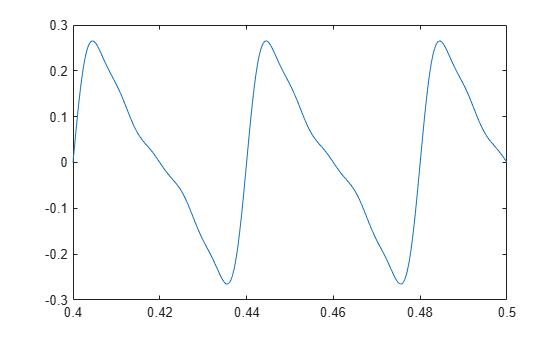A defect in the outer race of the bearing causes a series of 5 millisecond impacts on the bearing. Eventually, those impacts result in bearing wear. The impacts occur at the ball pass frequency outer race (BPFO) of the bearing,

`$BPFO=\frac{1}{2}n{f}_{0}\left[1-\frac{d}{p}\mathrm{cos}\theta \right],$`

where ${f}_{0}$ is the driving rate, n is the number of rolling elements, d is the diameter of the rolling elements, p is the pitch diameter of the bearing, and θ is the bearing contact angle. Assume a contact angle of zero and compute the BPFO.

```ca = 0; bpfo = n*f0/2*(1-d/p*cos(ca))```
```bpfo = 83.3333 ```

Model each impact as a 3 kHz sinusoid windowed by a flat top window. Make the impact periodic by convolving it with a comb function. Plot 0.1 second of data.

```fImpact = 3000; tImpact = 0:1/fs:5e-3-1/fs; xImpact = sin(2*pi*fImpact*tImpact).*flattopwin(length(tImpact))'/10; xComb = zeros(size(t)); xComb(1:fs/bpfo:end) = 1; x = conv(xComb,xImpact,'same')/3; plot(t,x+z) xlim([0.4 0.5])```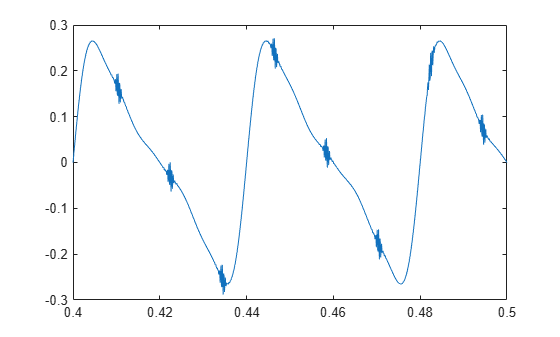Add white Gaussian noise to the signals. Specify a noise variance of 1/30². Plot 0.1 second of data.

```yGood = z + randn(size(z))/30; yBad = x+z + randn(size(z))/30; plot(t,yGood,t,yBad) xlim([0.4 0.5]) legend('Healthy','Damaged')```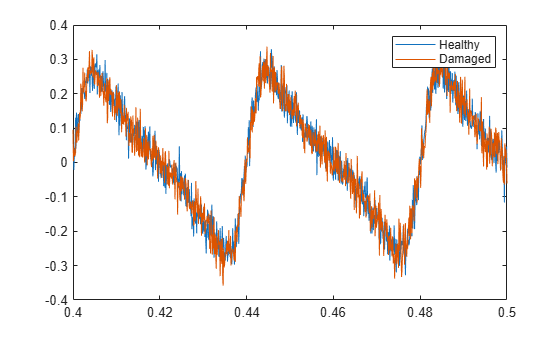Compute and plot the envelope signals and spectra.

```envspectrum([yGood' yBad'],fs) xlim([0 10*bpfo]/1000)```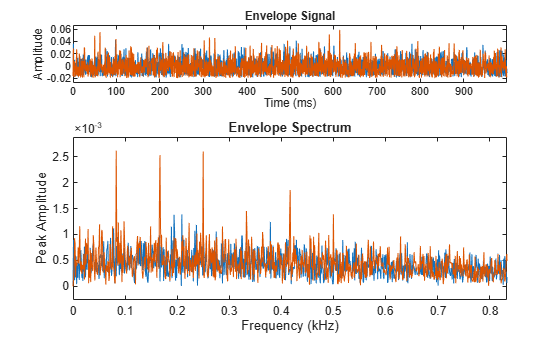Compare the peak locations to the frequencies of harmonics of the BPFO. The BPFO harmonics in the envelope spectrum are a sign of bearing wear.

```harmImpact = (1:10)*bpfo; [X,Y] = meshgrid(harmImpact,ylim); hold on plot(X/1000,Y,':k') legend('Healthy','Damaged','BPFO harmonics') hold off```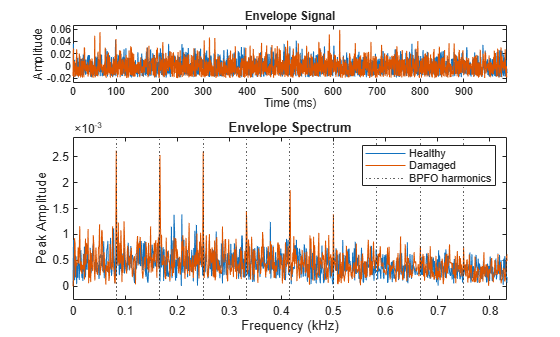Compute the Welch spectra of the signals. Specify a frequency resolution of 5 Hz.

```figure pspectrum([yGood' yBad'],fs,'FrequencyResolution',5) legend('Healthy','Damaged')```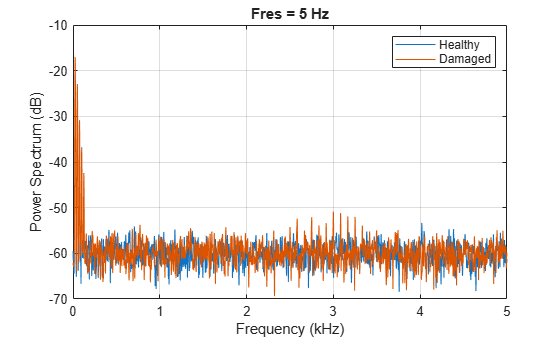At the low end of the spectrum, the driving frequency and its orders obscure other features. The spectrum of the healthy bearing and the spectrum of the damaged bearing are indistinguishable.

`xlim([0 10*bpfo]/1000)`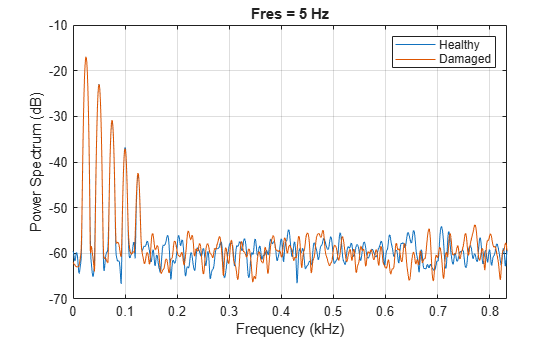The spectrum of the faulty bearing shows BPFO harmonics modulated by the impact frequency.

`xlim((bpfo*[-10 10]+fImpact)/1000)`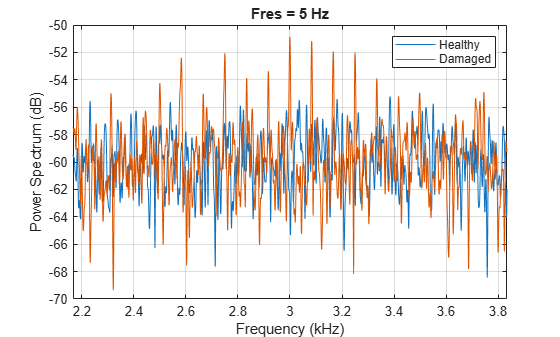Generate a two-channel signal that resembles the vibration signals from a bearing that completes a rotation every 10 milliseconds. The signal is sampled at 10 kHz for 0.2 seconds, which corresponds to 20 bearing rotations.

```fs = 10000; tmax = 20; mlt = 0.01; t = 0:1/fs:mlt-1/fs;```

During each 10-millisecond interval:

• The first channel is a damped sinusoid with damping constant 700 and sinusoid frequency 600 Hz.

• The second channel is another damped sinusoid with damping constant 800 and sinusoid frequency 500 Hz. The second channel lags the first channel by 5 milliseconds.

Plot the signal.

```y1 = sin(2*pi*600*t).*exp(-700*t); y2 = sin(2*pi*500*t).*exp(-800*t); y2 = [y2(51:100) y2(1:50)]; T = (0:1/fs:mlt*tmax-1/fs)'; Y = repmat([y1;y2],1,tmax)'; plot(T,Y)```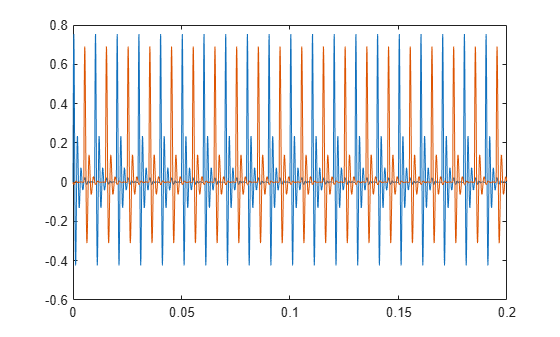Create a duration array using the time interval `T`. Construct a timetable with the duration array and the two-channel signal.

```dt = seconds(T); ttb = timetable(dt,Y);```

Use `envspectrum` with no output arguments to display the envelope signal and envelope spectrum of the two channels. Compute the spectrum on the whole Nyquist interval, excluding 100 Hz intervals at the ends.

`envspectrum(ttb,'Band',[100 4900])`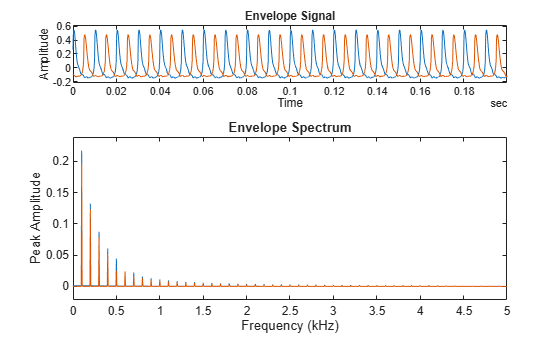The envelope spectra of the signals have peaks at integer multiples of the repetition rate of 1/0.01 = 0.1 kHz. This is just as expected. `envspectrum` removes the high-frequency sinusoidal components and focuses on the lower-frequency repetition behavior. This is why the envelope spectrum is a useful tool for the analysis of rotational machinery.

Compute the envelope signal and the times at which it is computed. Check the types of the output variables.

```[~,~,ttbenv,ttbt] = envspectrum(ttb,'Band',[100 4900]); whos ttb*```
``` Name Size Bytes Class Attributes ttb 2000x1 48977 timetable ttbenv 2000x1 48985 timetable ttbt 2000x1 16002 duration ```

The time vector is of `duration` type, like the time values of the input timetable. The output timetable has the same size as the input timetable.

Store each channel of the input timetable as a separate variable. Compute the envelope signal and the time vector. Check the output types.

```btb = timetable(dt,Y(:,1),Y(:,2)); [~,~,btbenv,btbt] = envspectrum(btb,'Band',[100 4900]); whos btb*```
``` Name Size Bytes Class Attributes btb 2000x2 49199 timetable btbenv 2000x2 49219 timetable btbt 2000x1 16002 duration ```

The output timetable has the same size as the input timetable.

Generate a signal sampled at 1 kHz for 5 seconds. The signal consists of 0.01-second rectangular pulses that repeat every T = 0.25 second. Amplitude modulate the signal onto a sinusoid of carrier frequency 150 Hz.

```fs = 1e3; tmax = 5; t = 0:1/fs:tmax; y = pulstran(t,0:0.25:tmax,'rectpuls',0.01); fc = 150; z = modulate(y,fc,fs);```

Plot the original and modulated signals. Show only the first few cycles.

```plot(t,y,t,z,'-') grid on axis([0 1 -1.1 1.1])```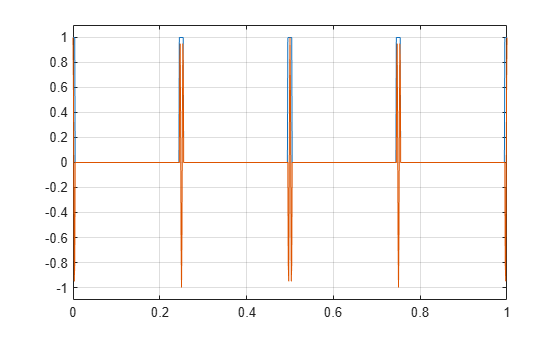Compute the envelope and envelope spectrum of the signal. Determine the signal envelope using complex demodulation. Compute the envelope spectrum on a 20 Hz interval centered at the carrier frequency.

`[q,f,e,te] = envspectrum(z,fs,'Method','demod','Band',[fc-10 fc+10]);`

Plot the envelope signal and the envelope spectrum. Zoom in on the interval from 0 to 50 Hz.

```subplot(2,1,1) plot(te,e) xlabel('Time') title('Envelope') subplot(2,1,2) plot(f,q) xlim([0 50]) xlabel('Frequency') title('Envelope Spectrum')```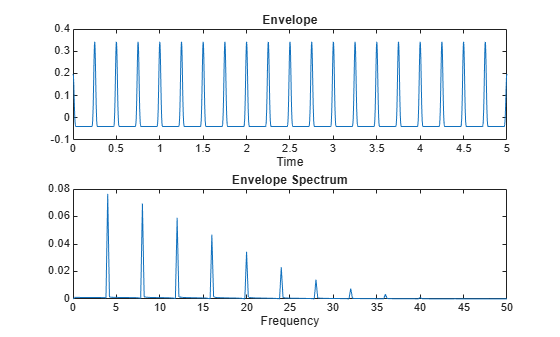The envelope signal has the same period in time, T = 0.25 second, as the original signal. The envelope spectrum has pulses at 1 / T = 4 Hz.

Repeat the computation, but now use the `hilbert` function to compute the envelope. Bandpass-filter the signal using a 10th-order finite impulse response (FIR) filter. Plot the envelope signal and envelope spectrum using the built-in functionality of `envspectrum`.

`envspectrum(z,fs,'Method','hilbert','FilterOrder',10)`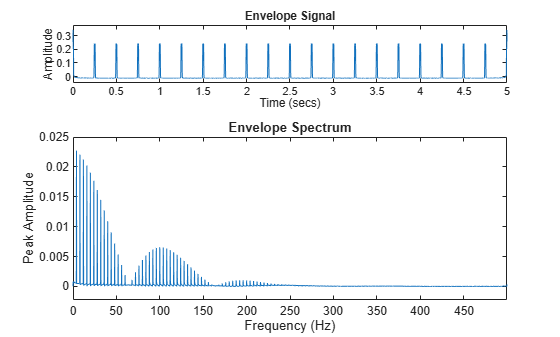Embed the signal in white Gaussian noise of variance 1/3. Plot the result.

```zn = z + randn(size(z))/3; plot(t,zn,'-') grid on axis([0 1 -1.1 1.1])```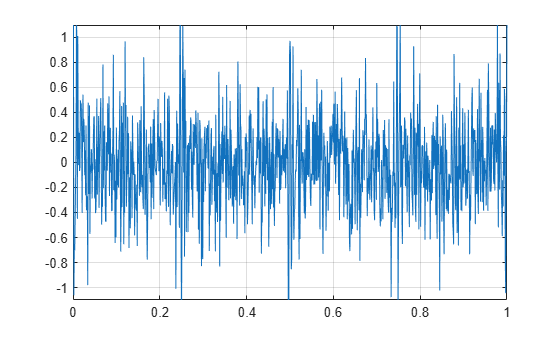Compute and display the envelope signal and envelope spectrum. Compute the envelope spectrum using complex demodulation on a 10 Hz interval centered at the carrier frequency. Zoom in on the interval from 0 to 50 Hz.

```envspectrum(zn,fs,'Band',[fc-5 fc+5]) xlim([0 50])```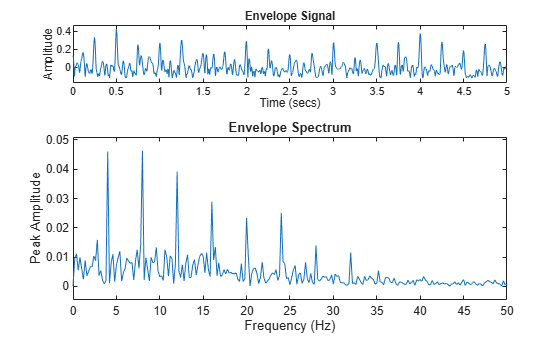## Input Arguments

collapse all

Input signal, specified as a vector or a matrix. If `x` is a vector, it is treated as a single channel. If `x` is a matrix, then `envspectrum` computes the envelope spectrum independently for each column and returns the result in the corresponding column of `es`.

Example: `cos(pi/4*(0:159))+randn(1,160)` is a single-channel row-vector signal.

Example: `cos(pi./[4;2]*(0:159))'+randn(160,2)` is a two-channel signal.

Data Types: `single` | `double`
Complex Number Support: Yes

Sample rate, specified as a positive real scalar.

Data Types: `single` | `double`

Input timetable. `xt` must contain increasing finite row times. If `xt` represents a multichannel signal, then it must have either a single variable containing a matrix or multiple variables consisting of vectors.

If a timetable has missing or duplicate time points, you can fix it using the tips in Clean Timetable with Missing, Duplicate, or Nonuniform Times.

Example: `timetable(seconds(0:4)',randn(5,2))` specifies a two-channel, random variable sampled at 1 Hz for 4 seconds.

Data Types: `single` | `double`

### Name-Value Pair Arguments

Specify optional comma-separated pairs of `Name,Value` arguments. `Name` is the argument name and `Value` is the corresponding value. `Name` must appear inside quotes. You can specify several name and value pair arguments in any order as `Name1,Value1,...,NameN,ValueN`.

Example: ```'Method','hilbert','FilterOrder',30,'Band',[0 fs/4]``` computes the envelope spectrum between 0 and one-half the Nyquist frequency using a 30th-order bandpass filter and computing the envelope of the analytic signal.

Algorithm for computing the envelope signal, specified as the comma-separated pair consisting of `'Method'` and either `'hilbert'` or `'demod'`. See Algorithms for more information.

Frequency band to compute envelope spectrum, specified as the comma-separated pair consisting of `'Band'` and a two-element vector of strictly increasing values between 0 and the Nyquist frequency.

Data Types: `single` | `double`
Complex Number Support: Yes

FIR filter order, specified as the comma-separated pair consisting of `'FilterOrder'` and a positive integer scalar.

• If `'Method'` is `'hilbert'`, then this argument specifies the order of an FIR bandpass filter.

• If `'Method'` is `'demod'`, then this argument specifies the order of an FIR lowpass filter.

Data Types: `single` | `double`

## Output Arguments

collapse all

Envelope spectrum, returned as a vector or matrix.

Frequencies at which the envelope spectrum is computed, returned as a vector.

Envelope signal, returned as a vector, matrix, or timetable.

If the input to `envspectrum` is a timetable, then `env` is also a timetable. The time values of `env` have the same format as the time values of the input timetable.

• If the input is a timetable with a single variable containing a matrix, then `env` has a single variable containing a matrix.

• If the input is a timetable with multiple variables consisting of vectors, then `env` has multiple variables consisting of vectors.

Time values at which the envelope signal is computed, returned as a vector.

If the input to `envspectrum` is a timetable, then `t` has the same format as the time values of the input timetable.

## Algorithms

`envspectrum` initially removes the DC bias from the input signal, `x`, and then computes the envelope signal.

• If `'Method'` is set to `'hilbert'`, the function:

1. Bandpass-filters the signal. The FIR filter has an order specified by `'FilterOrder'` and cutoff frequencies at `ba(1)` and `ba(2)`, where `ba` is a frequency band specified using `'Band'`.

2. Computes the analytic signal using the `hilbert` function.

3. Computes the envelope signal as the absolute value of the analytic signal.

• If `'Method'` is set to `'demod'`, the function:

1. Performs complex demodulation of the signal. The signal is multiplied by exp(j2πf0t), where f0 = (`ba(1)` + `ba(2)`)/2.

2. Lowpass-filters the demodulated signal to compute the analytic signal. The FIR filter has an order specified by `'FilterOrder'` and a cutoff frequency of (`ba(2)``ba(1)`)/2.

3. Computes the envelope signal as twice the absolute value of the analytic signal.

After computing the envelope signal, the function removes the DC bias from the envelope and computes the envelope spectrum using the FFT.

 Randall, Robert Bond. Vibration-Based Condition Monitoring. Chichester, UK: John Wiley & Sons, 2011.